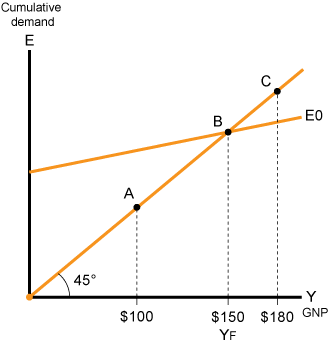# Keynes’ Theory Explained

## Every country can experience three different equilibrium situations.

1. Ideal equilibrium – in this situation, a country’s GDP is at the maximum, which is denoted as YF.

2. Equilibrium in an inferior situation (the usual term for this situation is deflationary gap). In this situation, equilibrium exists while GDP is less than the maximum.

3. Equilibrium in an impossible situation (the usual term for this situation is inflationary gap). In this situation, equilibrium exists although GDP is greater than the maximum. Equilibrium of this type exists only in diagrams, and not in reality.

Three situations relating to equilibrium:

Diagram 11.11

3 Situation of Equilibrium

Inflationary GapIdeal EquilibriumDeflationary Gap## Keynes’ Advice Concerning the Three Situations

1. Ideal Equilibrium

In this situation, there is full employment and no reason to adjust the situation.

2. Deflationary Gap

In this situation, unemployment exists and the economy is therefore not fully being exploited to its potential.

Keynes’ solution: Increase aggregate demand from E0 to E1 (the broken line in the diagram) and then the equilibrium will be ideal. Aggregate demand can be increased through some or all of the demand factors, which are as follows:

1. Private consumption.

2. Public consumption.

3. Investment.

Deflationary gap